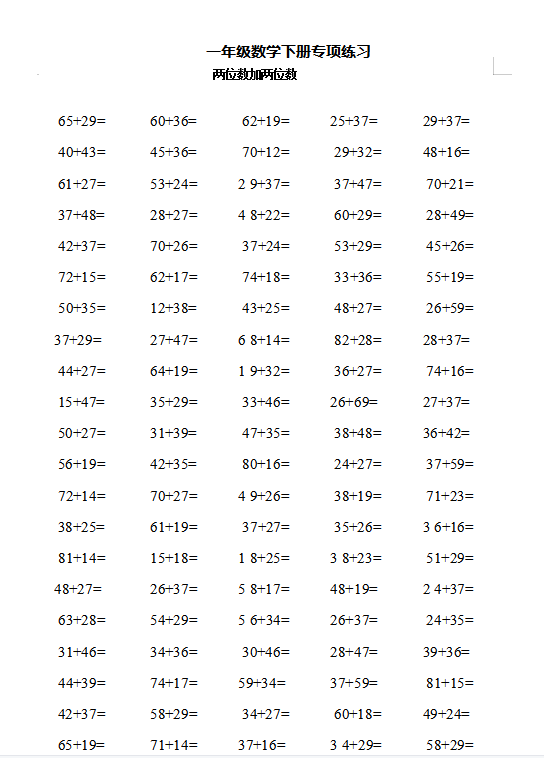65+29=
40+43=
61+27=
37+48=
42+37=
72+15=
50+35=
37+29=
44+27=
15+47=
50+27=
56+19=